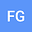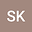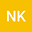Transition chaos in fractional order Cournot duopoly game model on scale-free network
•••• Fuat Gurcan,
• Şenol KARTAL,
• Neriman Kartal
Fuat Gurcan
Kuwait University

Corresponding Author:[email protected]

Author ProfileŞenol KARTAL
Nevsehir Haci Bektas Veli Universitesi
Author ProfileNeriman Kartal
Nevsehir Haci Bektas Veli Universitesi
Author Profile## Abstract

In this study, an Cournot duopoly model describing conformable fractional order differential equations with piecewise constant arguments is discussed. We have obtained two dimensional discrete dynamical system as a result of the discretization process is applied to the model. By using the center manifold theorem and the bifurcation theory, it is shown that the discrete dynamical system undergoes flip bifurcation about the Nash equilibrium point. Phase portraits, bifurcation diagrams, Lyaponov exponents show the existence of many complex dynamical behavior in the model such as stable equilibrium point, period-2 orbit, period-4 orbit, period-8 orbit, period-16 orbit and chaos according to changing the speed of adjustment parameter v 1 . Discrete Cournot duopoly game model is also considered on a Scale free network with N=10 and N=100 nodes. It is observed that the complex dynamical network exhibits similar dynamical behavior such as stable equilibrium point, Flip bifurcation and chaos depending on the changing the coupling strength parameter c s . Moreover, flip bifurcation and transition chaos happen earlier in more heterogeneous networks. Calculating the Largest Lyapunov exponents guarantee the transition from nonchaotic to chaotic states in complex dynamical networks.